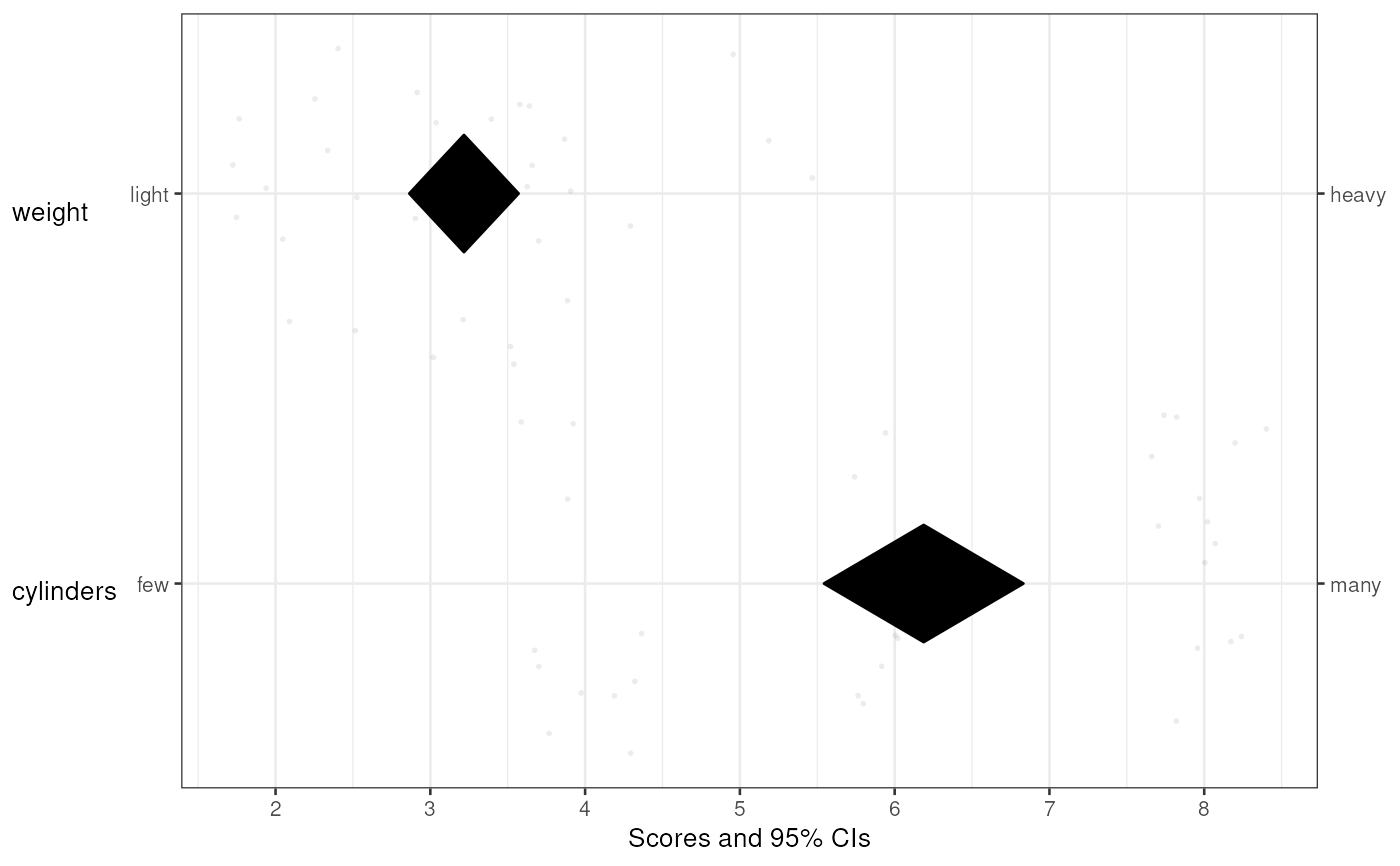This is basically a meansDiamondPlot(), but extended to allow specifying subquestions and anchors at the left and right side. This is convenient for psychological questionnaires when the anchors or dimensions were different from item to item. This function is used to function the left panel of the CIBER plot in the behaviorchange package.

biAxisDiamondPlot(
dat,
items = NULL,
leftAnchors = NULL,
rightAnchors = NULL,
subQuestions = NULL,
decreasing = NULL,
conf.level = 0.95,
showData = TRUE,
dataAlpha = 0.1,
dataColor = "#444444",
diamondColors = NULL,
jitterWidth = 0.45,
jitterHeight = 0.45,
xbreaks = NULL,
xLabels = NA,
xAxisLab = paste0("Scores and ", round(100 * conf.level, 2), "% CIs"),
drawPlot = TRUE,
returnPlotOnly = TRUE,
baseSize = 1,
dotSize = baseSize,
baseFontSize = 10 * baseSize,
theme = ggplot2::theme_bw(base_size = baseFontSize),
outputFile = NULL,
outputWidth = 10,
outputHeight = 10,
ggsaveParams = ufs::opts\$get("ggsaveParams"),
...
)

## Arguments

dat

The dataframe containing the variables.

items

The variables to include.

leftAnchors

The anchors to display on the left side of the left hand panel. If the items were measured with one variable each, this can be used to show the anchors that were used for the respective scales. Must have the same length as items.

rightAnchors

The anchors to display on the left side of the left hand panel. If the items were measured with one variable each, this can be used to show the anchors that were used for the respective scales. Must have the same length as items.

subQuestions

The subquestions used to measure each item. This can also be used to provide pretty names for the variables if the items were not measured by one question each. Must have the same length as items.

decreasing

Whether to sort the items. Specify NULL to not sort at all, TRUE to sort in descending order, and FALSE to sort in ascending order.

conf.level

The confidence levels for the confidence intervals.

showData

Whether to show the individual datapoints.

dataAlpha

The alpha level (transparency) of the individual datapoints. Value between 0 and 1, where 0 signifies complete transparency (i.e. invisibility) and 1 signifies complete 'opaqueness'.

dataColor

The color to use for the individual datapoints.

diamondColors

The colours to use for the diamonds. If NULL, the generateColors argument can be used which will then be passed to diamondPlot().

jitterWidth

How much to jitter the individual datapoints horizontally.

jitterHeight

How much to jitter the individual datapoints vertically.

xbreaks

Which breaks to use on the X axis (can be useful to override ggplot()'s defaults).

xLabels

Which labels to use for those breaks (can be useful to override ggplot()'s defaults; especially useful in combination with xBreaks of course).

xAxisLab

Axis label for the X axis.

drawPlot

Whether to draw the plot, or only return it.

returnPlotOnly

Whether to return the entire object that is generated (including all intermediate objects) or only the plot.

baseSize

This can be used to efficiently change the size of most plot elements.

dotSize

This is the size of the points used to show the individual data points in the left hand plot.

baseFontSize

This can be used to set the font size separately from the baseSize.

theme

This is the theme that is used for the plots.

outputFile

A file to which to save the plot.

outputWidth, outputHeight

Width and height of saved plot (specified in centimeters by default, see ggsaveParams).

ggsaveParams

Parameters to pass to ggsave when saving the plot.

...

These arguments are passed on to diamondPlot].

## Value

Either just a plot (a gtable::gtable() object) or an object with all produced objects and that plot.

## Details

This is a diamondplot that can be used for items/questions where the anchors of the response scales could be different for every item. For the rest, it is very similar to meansDiamondPlot().

CIBER() in the behaviorchange package, associationsDiamondPlot()

## Author

Gjalt-Jorn Peters

Maintainer: Gjalt-Jorn Peters gjalt-jorn@userfriendlyscience.com

## Examples


biAxisDiamondPlot(dat=mtcars,
items=c('cyl', 'wt'),
subQuestions=c('cylinders', 'weight'),
leftAnchors=c('few', 'light'),
rightAnchors=c('many', 'heavy'),
xbreaks=0:8);